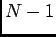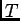# OOF: Finite Element Analysis of Microstructures

ASCII FormatNext: Binary Format Up: Data File Formats Previous: Data File Formats   Contents

Subsections

## ASCII Format

The ASCII data file is read and processed by the same command parser that is used for OOF's text interface (Section 6.1). This allows some flexibility in formatting. However, it is recommended that you stick with the format described below.

An ASCII data file begins with the line

```version number = 5
```
The version number may change in future releases. This manual describes only version 5.

The next two lines specify the number of elements and nodes in the grid.

```Nelements = <n>
Nnodes = <n>
```
If these lines are omitted, or if the numbers are wrong, the program will still work, but memory will not be used efficiently.

There are five additional sections to the data file: nodes, elements, nodegroups, elementgroups, and startup commands. Nodes must precede elements, and both must precede the other three sections.

In what follows, quantities printed in angle brackets like <this> are values to be supplied by the user.

### Nodes

Begin this section with the line:

```nodes (
```
Each node in the grid is described by a single line of the form:
```<name> i=<i> x=<x> y=<y> dx=<dx> dy=<dy> <etc>
```

<i> is the index of the node. Each node must have a unique non-negative integer index. The indices don't have to be in order, but all integers between 0 andmust be present, whereis the number of nodes. <x> and <y> are the initial position of the node. <dx> and <dy> are its displacement from its initial position.

The values to be used for <name> and <etc> depend on the type of node (see Section 3.13.2).

For xy nodes, <name> is xy and <etc> is blank.

For linear nodes, <name> is linear, and <etc> is the matrixthat transforms a 2-vector in the node's coordinate system into the OOF coordinate system.is specified as T00=<t00> T01=<t01> T10=<t10> T11=<t11>.

Close the node section with a closing parenthesis:

```)
```

### Elements

Begin this section with the line:

```elements (
```
Each element in the grid is described by a single line of the form:
```<name> i=<i> n1=<n1> n2=<n2> n3=<n3> gray=<g> <etc>
```

<name> is the name of the element type, as it appears in the menus (see Section 4.5). <i> is a unique element index. Like the node indices, the element indices don't have to be in order, but there can be no missing indices in the data file. <n1>, <n2>, and <n3> are the indices of the nodes at the corners of the element, going counterclockwise around the element. <g> is the element's gray value.

The data in <etc> depends on the element type. It consists of all the element's parameters, as listed in Section 4.5, in the format <name>=<value>. Remember that all elements have a gray parameter, and all but the empty elements have a planestrain parameter.

For example, an isotropic element might look like:

```isotropic i=7 n1=4 n2=25 n3=24 gray=0.5
poisson=0 young=1 alpha=0 planestrain=false
```
(all typed on one line, though). A hexagonal element might look like:
```hexagonal i=252 n1=132 n2=133 n3=153 gray=0.75 orientation=[10, 90, 0]
planestrain=false c11=1 c12=0 c13=0 c33=1 c44=0.5 alpha11=1 alpha33=1
```
and an empty element might look like:
```empty i=533 n1=280 n2=301 n3=300 gray=1
```

Elements that have mutated (3.1.4) will have extra (as yet undocumented) parameters to describe the mutation. It is unlikely that users would want to write .goof files with mutated elements.

Close the element section with a closing parenthesis:

```)
```

### Node Groups

If there are any node groups, they should be listed next. The format is:

```nodegroup (
label=<group name>
node=<i>
...
)
```
where <i> is the index of a node in the group. The ... means repeat the node= line for each node in the group.

### Element Groups

Element groups can be listed before or after the node groups. The format is very similar to the node group format:
```elementgroup (
label=<group name>
elem=<i>
...
)
```

### Startup Commands

Any OOF commands at the end of the file will be executed as they are encountered. This lets boundary conditions, distortions, etc, be set automatically. These commands, however, must be preceded by the word oof, indicating that they come from the main OOF menu, and not from the menu that's used to load the nodes and elements. For example, to set fixed boundary conditions on the top and bottom node groups, put the lines

```oof bc fix both top
oof bc fix both bottom
```
at the end of the .goof file. For more on the text versions of OOF commands, see Section 6.1.Next: Binary Format Up: Data File Formats Previous: Data File Formats   Contents
/* Send mail to the OOF Team *//* Go to the OOF Home Page */